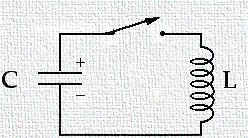### Circuit Theory 2b - Problems related to RL, LC, RLC Circuits and related Oscillations## LC and RLC Circuits and the oscillations associated with them.

### LC CircuitsC and L elements.

U = Uc + UL

When the capacitor is fully discharged, there is zero energy stored in it. At this moment, the current reaches its maximum value, and all of the energy is transformed into magnetic field and is stored in the inductor. Now, the current continues in the same direction, decreasing in magnitude, with the inductor getting discharged and eventually capacitor becoming fully charged. But the polarity of capacitor plates is now opposite to the initial polarity. This is followed by another discharge until the circuit returns to its original state of maximum charge Q and the plate polarity

The oscillations of the LC circuit are analogous to the mechanical oscillations of the spring mass system. Many of those concepts are applicable to LC oscillations. We will show how that the angular frequency of the oscillations depends only on the inductance and capacitance of the circuit. As the Q is varying sinusoidally , the current in the circuit also varies sinusoidally.
During oscillation of energy, the same energy is being oscillated between both the elements satisfying energy conservation.

Similarly, we will also cover RLC Circuits.

### RLC series circuit

We now consider a practical circuit consisting of an inductor, capacitor and a resistor connected in series. Let us assume that the initial charge on capacitor is Qmax before the switch is closed. Once the switch is closed and a current is set up, the total energy stored in the capacitor and inductor can be found out by previously derived equations. But in this case, the total energy of the circuit is not constant, like it was in LC circuit. This is because of the resistor element in the circuit, which causes energy dissipation across it in for of heat and radiations. As the energy loss across the resistance is given by -I2

We explain how to compute the current (as a function of time), the damping factor and the angular frequency of the damped oscillations. We also illustrate the analogies between electrical and mechanical systems..

#### A quick look at the nature of questions/problems solved in this tutorial:

• In a given figure, the switch K is kept first at position 1 and steady state condition is reached. At t=0, the switch is moved to position 2. Find the current in both the cases.
• In a given figure steady state condition is reached with 100V d.c. source. At t=0, switch K is suddenly opened. Find the  expression of the current through the inductor after t=0.5sec.
• In a given figure, the switch is closed at position 1 at t=0. At t=0.5 msec. the switch is moved to position 2. Find the expression  for the current in both the conditions.
• In a given figure a d.c. voltage of 100v is applied in the circuit and the switch K is open. the switch k is closed at t=0. Find the complete expression for the current.
• You will learn to apply KVL and KCL on a variety of circuits, frame differential equations; use basic concepts of differential and integral calculus to obtain a solution.
• Steady state analysis of circuits containing resistors, inductance and capacitors.

### Complete tutorial with solved problems :

#### MCQ: DC Circuits with R/L/C

Related Tutorials ( Introduction to Electrical Circuits - DC ) :

 Circuit Theory 1a - Introduction to Electrical Engineering, DC Circuits, Resistors, Capacitors, problems related to these. Circuit Theory 1b - More solved problems related to DC Circuits with Resistance and Capacitance Capacitors, computing capacitance, RC Circuits, time constant of decay, computing voltage and electrostatic energy across a capacitance Circuit Theory 2a - Introducing Inductors Inductors, inductance, computing self-inductance, flux-linkages, computing energy stored as a magnetic field in a coil,  mutual inductance, dot convention, Circuit Theory 2b - Problems related to RL, LC, RLC circuits Introducing the concept of oscillations. Solving problems related to RL, LC and RLC circuits using calculus based techniques. Circuit Theory 3a - Electrical Networks and Network Theorems Different kind of network elements: Active and passive, linear and non-linear, lumped and distributed. Voltage and current sources. Superposition theorem, Thevenin (or Helmholtz) theorem and problems based on these. Circuit Theory 3b - More network theorems, solved problems More solved problems and examples related to electrical networks. Star and Delta network transformations, maximum power transfer theorem, Compensation theorem and Tellegen's Theorem and examples related to these.